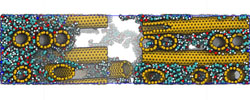### Other

Teacher AssistantGraphic Processor Units for Many-particle Dynamics

.This course describes, besides the physical and mathematical background of the finite element method, special discretization techniques and algorithms which have to be applied for nonlinear problems of solid mechanics.

The course will describe the theory and computer implementation of nonlinear finite element method. The implementation will be carried out on simple heat transfer and solid mechanics problems.

The focus is on geometric nonlinearities; however some material nonlinearity will also be discussed. Both static and transient (time-dependent) problems will be studied.

The main textbook of the course is the book “An Introduction to nonlinear finite element analysis” by J.N. Reddy. The book is suitable as a text book for a first course as nonlinear finite elements in mechanical, civil, aerospace engineering departments.

Syllabus

 Introduction A review of finite element method 1D problems 2D problems Library of 2D finite elements Numerical Integration Computer Implementation 1D heat transfer problems Governing differential equation Weak formulation Finite element model Solution procedure Computer implementation Euler-Bernouli beams Timoshenko beams Model equation Weak form Finite element method Solution procedure Computer implementation Classical plate theory (CPT) Variational formulation of CPT Finite element models of CPT Computer Implementation of CPT Time approximation Stability and accuracy Transient analysis of nonlinear problems Computer implementation Numerical examples 2D Continuum Elements Introduction Strain and stress measures Strain and stress measures between configurations Constitutive equations Total and updated Lagrangian formulations Finite element methods of 2D continua Nonlinear elastic problems Small deformation theory of plasticity Numerical examples Nonlinear bending of beams 2D heat transfer problems Nonlinear bending of elastic plates Nonlinear analysis of 2D problems Finite element formulation of solid continua Material nonlinearity

Prerequisites:

• Finite Element I
• Continuum Mechanics

### Random Images### Visitors Counter

We have 75 guests and no members online

### Latest News

#### Unified Memory in CUDA 6

With CUDA 6, we’re introducing one of the most dramatic programming model improvements in the history of the CUDA platform, Unified Memory. In a typical PC or cluster node today, the memories of the CPU and GPU are physically distinct and separated by the PCI-Express bus. Before CUDA 6, that is exactly how the programmer has to view things. Data that is shared between the CPU and GPU must be allocated in both memories, and explicitly copied between them by the program. This adds a lot of complexity to CUDA programs.